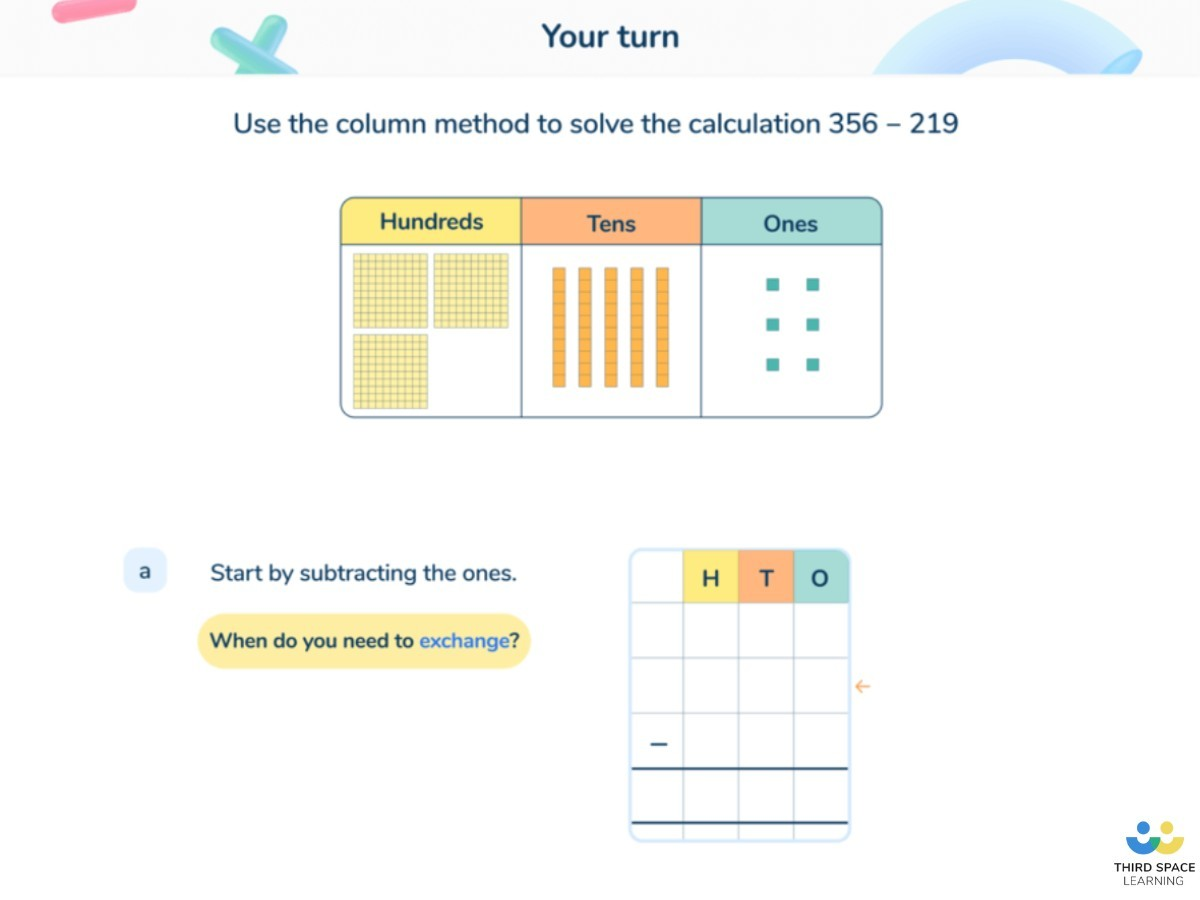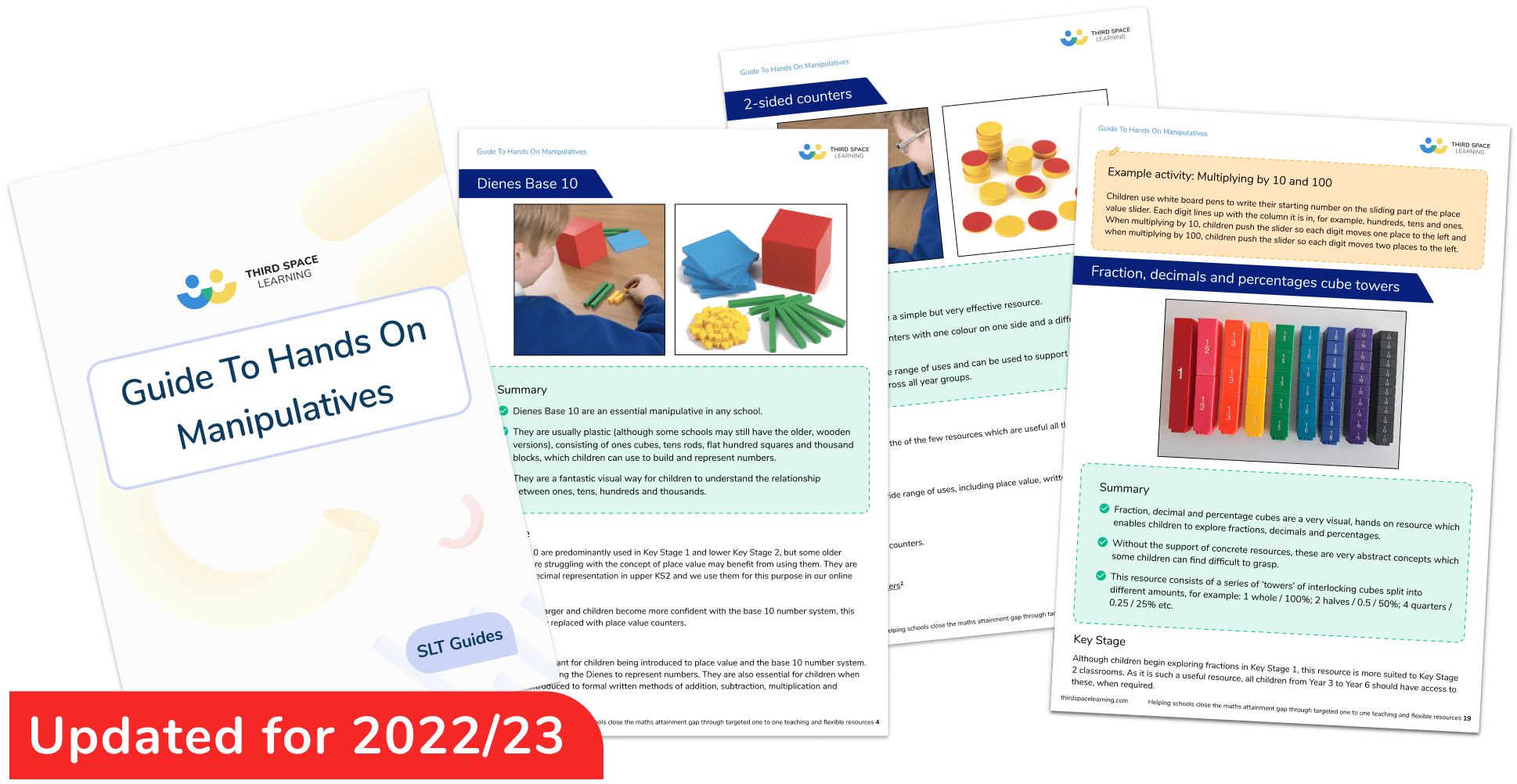Teaching support from the UK’s largest provider of in-school maths tuitionone to one lessonsschools supported

Built by teachers for teachers

In-school online one to one maths tuition developed by maths teachers and pedagogy experts

Find out more

Hundreds of FREE online maths resources!

Daily activities, ready-to-go lesson slides, SATs revision packs, video CPD and more!Explore all resources
Maths Mastery

Built by teachers for teachers

In-school online one to one maths tuition developed by maths teachers and pedagogy experts

Find out more

# What Are Dienes? Explained For Primary Schools

Dienes are a versatile addition to the primary maths classroom. As more schools adopt a maths mastery approach to teaching maths, interest has grown in the use of concrete resources and maths manipulatives to introduce and explore maths topics.

Dienes – also known as base ten – are versatile manipulatives which allow teachers and students to represent and understand numbers. They can be used to teach a large proportion of the maths curriculum including place value, calculation and decimals throughout the primary age range. In this blog, we’ll give you an introduction to Dienes and their use in the primary setting and provide you with practice questions and worked examples that could be answered using Dienes.

### What are Dienes?

Dienes are coloured plastic or wooden blocks that are used to represent numbers. There are four different Dienes blocks. They are usually used to represent 1000, 100, 10 and 1.

• Dienes are proportionally correct. Unlike place value counters or Cuisenaire rods, Dienes are proportionally correct. This means ten ‘ones’ cubes are the same size as one ‘ten’ rod and this continues with each larger piece. This allows children to understand the relationship between the different columns on a place value grid.
• Dienes can be used to assist with regrouping and exchanging in addition and subtraction. Whilst they are commonly used to portray (up to) four digit numbers, the proportional nature of Dienes also allow teachers to use them to show decimal numbers and their place value. This is particularly useful when first introducing decimals in lower Key Stage 2.
• Dienes are easy for children to use pictorially. They can quickly and neatly draw Dienes to either help them calculate answers or to prove their work.In our one to one tuition, we use pictorial representations of Dienes to support pupils, as in this column subtraction example.Guide To Hands On Manipulatives

A guide to 15 hands on resources every primary classroom should have - and how to get them on a budgetDownload Free Now!

### Why are they called Dienes?

Dienes are named after a Hungarian mathematician Zoltán Pál Dienes who famously theorised that the best way for children, from Early Years and beyond, to learn maths is through games, songs and dance to make it more appealing and memorable. He is credited with inventing the base ten block.

### How are Dienes used in maths?

As they are so versatile, Dienes blocks can be used to enhance pupils’ understanding and reasoning. They have become a staple teaching resource in many mathematics classrooms across the country to enable pupils to master the curriculum.

#### Dienes for place value

It is fundamental that children understand place value to access the rest of the mathematics curriculum. Every academic year usually begins with pupils revisiting place value in the spiral curriculum. Dienes can help pupils to visualise the numbers that they are working with.

For example, Year 2 pupils can use Dienes blocks to represent a two-digit number and explain how many tens and ones in a given number.

You could stretch pupils further by asking, ‘Do these two representations show the same number?’

In this example, a) shows 3 tens and 2 ones which is 32 and b) shows 2 tens and 12 ones which is also 32. Pupils may initially disagree that they are the same as there are a different number of tens, however understanding that these representations are two different ways of showing the same number will help with addition and subtraction later on.

##### Read more: What Is A Rekenrek? and 100 Square Activities For Primary School

Once pupils are comfortable using Dienes to show the place value of numbers and manipulate these to create different representations, they can begin to use them to calculate.

An advantage of Dienes over resources such as number lines or counting materials is that they can be used for three- and four-digit numbers without becoming confusing or time consuming for pupils to count.

Dienes can be used alongside a written calculation in column addition to show how the calculation would be set out and to help pupils visualise the numbers that they are working with, as shown below.

As long as pupils understand which Dienes represent hundreds, tens and ones, it is as easy as counting in multiples of that number.

Having learnt column addition without regrouping, pupils can also use Dienes to understand how to cross the tens line. This is where being able to represent numbers in more than one way becomes useful.

#### Dienes for subtraction

In a similar fashion, Dienes blocks can be used to teach subtraction too, both as a stand alone resource to complete calculations and alongside column subtraction.

Again, once pupils are comfortable subtracting without regrouping and exchanging, they can then exchange larger Dienes blocks for ten of the smaller ones in order to be able to complete subtraction calculations with regrouping and exchanging.

#### Dienes for decimals

Dienes can also be used to help pupils to visualise decimals. Until this point, we have been using Dienes to represent the same numbers every time. However, since they are just within a power of ten of one another, it is possible to scale their values down to represent tenths, hundredths and thousandths.

At this stage, pupils will have gained familiarity using Dienes which will help them to understand the place value of decimal numbers when this is introduced in Year 4.

From here, teaching addition and subtraction of decimals and their relationships to fractions (and later percentages) can follow the same pattern as with whole numbers as discussed earlier.

### When do children use Dienes in school?

In primary school, pupils can use Dienes blocks right from Early Years, when they are first discovering and understanding number, through to Year 6 when they are working with fractions, decimal numbers and high-level addition and subtraction. Similarly, they can be used to help children with fluency in calculation and to reason and problem solve. Children can use Dienes blocks to prove their answers to a question, as well as represent numbers.

Aside from helping children to understand the number system and how numbers relate to one another, Dienes can help children to develop skills for their lives outside the classroom. Most notably, they allow children to develop problem solving and reasoning skills which they can then apply to their wider lives.

### Dienes worked examples

1. What number is represented?

This is completed by counting the tens and ones.

Pupils could then be extended by asking them to show this in a different way (maybe 7 tens and 17 ones or with a different resource, such as place value counters)

2. Complete the part-whole model using Dienes.

This is drawn as if they were the children’s jottings to record the Dienes.

There are multiple different answers to this problem, as long as the drawn Dienes total 350, since there are already 15 ‘ones’ shown.

A potential misconception here will be to immediately draw 300 and 60.

3. Fill in the missing number

Again, I have completed this using both the jottings and column addition, as you may expect pupils to.

Emphasise that we begin on the right to ensure that if we need to exchange, we can.

4. 637 – 184

In this calculation, I have set out the column subtraction with the Dienes pictorially, just as I would if I were teaching it to pupils with the concrete resource.

The question has provided the Dienes here, but the question could just give the calculation and expect the children to draw the Dienes themselves.

I began on the right as you would for column subtraction and I noticed that an exchange needed to be completed which I have shown in my working out.

5. Which decimal number is represented?

6. Year 4 are fundraising for charity. Their target is £3250.

Class A raised £1124, Class B raised £952.

How much does Class C need to raise?

First, I have added £1124 and £952 using the Dienes. This will tell me how much has already been raised.

Then, I need to subtract my answer from the target – £3250.

The answer to the question is that Class C needs to raise £1174.

### Dienes practice questions

1. What number is represented? How could you represent the same number in another way?

Children could also show this with 50+13, 40+23. Encourage them to not go too much further as it becomes difficult to count. Other options include bar models, part-whole models, place value counters or number lines.

2. Complete the part-whole model using Dienes.

Answer: Any combination which makes 4503. Discourage children from drawing 503 ones (or similar) as this is not helpful and wastes time.

3. Find the missing number

4. 561 – 324

5. Which decimal number is represented?

6) There are 5832 seats in a stadium.

The home team have sold 3418 tickets and the away team have sold 833.

How many tickets are left?

What are Dienes in maths?

Dienes are a versatile manipulative resource which can be used to represent numbers.

Are Dienes and Base 10 the same?

Yes. Base 10 are the blocks that Professor Zoltan Dienes produced to help children understand the number system. We sometimes use his name for the same materials.

Why are Dienes blocks good?

Dienes blocks have multiple uses in every primary classroom. All children can use them to represent numbers in their place value units, but they can also be used for addition, subtraction and decimals.

How do you subtract with Dienes?

To subtract with Dienes, first make the number that you are subtracting from (the minuend) out of Dienes. Next, physically remove the number that you are subtracting (the subtrahend), remembering to begin on the right in case any exchanges are needed. The answer is the Dienes that you have left.

Do you have pupils who need extra support in maths?
Every week Third Space Learning’s maths specialist tutors support thousands of pupils across hundreds of schools with weekly online 1-to-1 lessons and maths interventions designed to plug gaps and boost progress.

Since 2013 we’ve helped over 150,000 primary and secondary school pupils become more confident, able mathematicians. Learn more or request a personalised quote for your school to speak to us about your school’s needs and how we can help.

Subsidised one to one maths tutoring from the UK’s most affordable DfE-approved one to one tutoring provider.##### Lauren Marlow
Lauren is a primary school teacher, maths mastery specialist and former maths lead who teaches in schools across Leicestershire, alongside writing blogs and resources for Third Space Learning.

Related Articles

x#### Guide To Hands On Manipulatives

A guide to 15 hands on resources every primary classroom should have - and how to get them on a budget

#### Guide To Hands On ManipulativesA guide to 15 hands on resources every primary classroom should have - and how to get them on a budget